# OpenGL ES Pixel Shaders Tutorial

In this OpenGL ES pixel shaders tutorial, take a deep dive into GLSL and fragment shader math – including how to make gradients and random noise!

5/5 3 Ratings

#### Version

• Other, Other, Other

In this pixel shaders tutorial, you’ll learn how to turn your iPhone into a full-screen GPU canvas.

What this means is that you’ll make a low-level, graphics-intensive app that will paint every pixel on your screen individually by combining interesting math equations.

But why? Well, besides being the absolute coolest things in computer graphics, pixel shaders can be very useful in:

Note: The demos linked above use WebGL, which is only fully supported on Chrome and Opera, at least at the time of writing this tutorial. These demos are also pretty intense – so try to have them not running on multiple tabs simultaneously.

The shaders you’ll write are not as complex as the ones above, but you’ll get a lot more out of these exercises if you’re familiar with OpenGL ES. If you’re new to the API, then please check out some of our written or video tutorials on the subject first :]

Without further ado, it is my pleasure to get you started with pixel shaders in iOS!

Note: The term “graphics-intensive” is no joke in this tutorial. This app will safely push your iPhone’s GPU to its limit, so use an iPhone 5 or newer version. If you don’t have an iPhone 5 or later, the iOS simulator will work just fine.

## Getting Started

First, download the starter pack for this tutorial. Have a look at `RWTViewController.m` to see the very light `GLKViewController` implementation, and then build and run. You should see the screen below: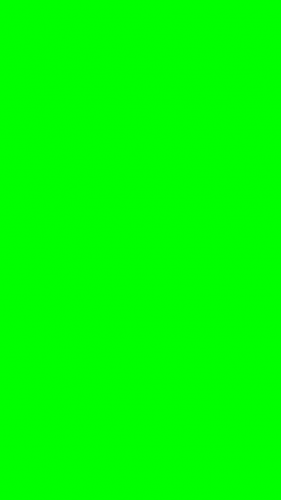Nothing too fancy just yet, but I’m sure Green Man would approve :]

For the duration of this tutorial, a full green screen means your base shaders (`RWTBase.vsh` and `RWTBase.fsh`) are in working order and your OpenGL ES code is set up properly. Throughout this tutorial, green means “Go” and red means “Stop”.

If at any point you find yourself staring at a full red screen, you should “Stop” and verify your implementation, because your shaders failed to compile and link properly. This works because the `viewDidLoad` method in RWTViewController sets `glClearColor()` to red.

A quick look at RWTBase.vsh reveals one of the simplest vertex shaders you’ll ever encounter. All it does is calculate a point on the x-y plane, defined by `aPosition`.

The vertex attribute array for `aPosition` is a quad anchored to each corner of the screen (in OpenGL ES coordinates), named `RWTBaseShaderQuad` in `RWTBaseShader.m`. RWTBase.fsh is an even more simple fragment shader that colors all fragments green, regardless of position. This explains your bright green screen!

Now, to break this down a bit further…

If you’ve taken some of our previous OpenGL ES tutorials, you may have noticed that we talk about vertex shaders for manipulating vertices and fragment shaders for manipulating fragments. Essentially, a vertex shader draws objects and a fragment shader colors them. Fragments may or may not produce pixels depending on factors such as depth, alpha and viewport coordinates.

So, what happens if you render a quad defined by four vertices as shown below?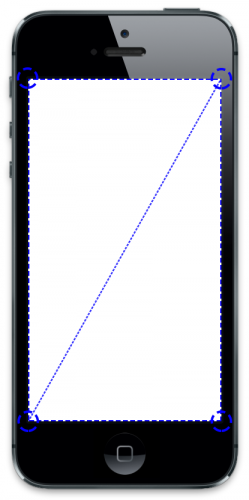Assuming you haven’t enabled alpha blending or depth testing, you get an opaque, full-screen cartesian plane.

Under these conditions, after the primitive rasterizes, it stands to reason that each fragment corresponds to exactly one pixel of the screen – no more, no less. Therefore, the fragment shader will color every screen pixel directly, thus earning itself the name of pixel shader :O

Note: By default, `GL_BLEND` and `GL_DEPTH_TEST` are disabled. You can see a list of `glEnable()` and `glDisable()` capabilities here, and you can query them programmatically using the function `glIsEnabled()`.

Note: In order to conserve space and focus on the algorithms/equations presented in this tutorial, the global GLSL `precision` value for `floats` is defined as `highp`.

The official OpenGL ES Programming Guide for iOS has a small section dedicated to precision hints which you can refer to afterwards for optimization purposes, along with the iOS Device Compatibility Reference.

Remember, for a full-screen iPhone 5, each fragment shader gets called 727,040 times per frame! (640*1136)

The magic behind pixel shaders lies within `gl_FragCoord`. This fragment-exclusive variable contains the window-relative coordinates of the current fragment.

For a normal fragment shader, “this value is the result of fixed functionality that interpolates primitives after vertex processing to generate fragments”. For pixel shaders, however, just know the `xy` swizzle value of this variable maps exactly to one unique pixel on the screen.

Open `RWTGradient.fsh` and add the following lines just below precision:

```// Uniforms
uniform vec2 uResolution;
```

`uResolution` comes from the `rect` variable of `glkView:drawInRect:` within `RWTViewController.m` (i.e. the rectangle containing your view).

`uResolution` in `RWTBaseShader.m` handles the width and height of `rect` and assigns them to the corresponding GLSL uniform in the method `renderInRect:atTime:`. All this means is that `uResolution` contains the x-y resolution of your screen.

Many times you’ll greatly simplify pixel shader equations by converting pixel coordinates to the range `0.0 ≤ xy ≤ 1.0`, achieved by dividing `gl_FragCoord.xy/uResolution`. This is a perfect range for `gl_FragColor` too, so let’s see some gradients!

Add the following lines to `RWTGradient.fsh` inside `main(void)`:

```vec2 position = gl_FragCoord.xy/uResolution;
gl_FragColor = vec4(0., gradient, 0., 1.);
```

Next, change your program’s fragment shader source from `RWTBase` to `RWTGradient` in `RWTViewController.m` by changing the following line:

```self.shader = [[RWTBaseShader alloc] initWithVertexShader:@"RWTBase" fragmentShader:@"RWTBase"];
```

to:

```self.shader = [[RWTBaseShader alloc] initWithVertexShader:@"RWTBase" fragmentShader:@"RWTGradient"];
```

Build and run! Your screen should show a really nice black->green gradient from left->right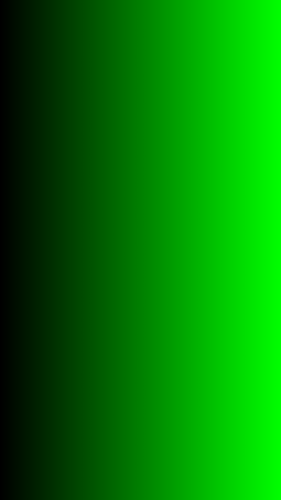Pretty cool, eh? To get the same gradient from bottom->top, change the following line in `RWTGradient.fsh`:

```float gradient = position.x;
```

to:

```float gradient = position.y;
```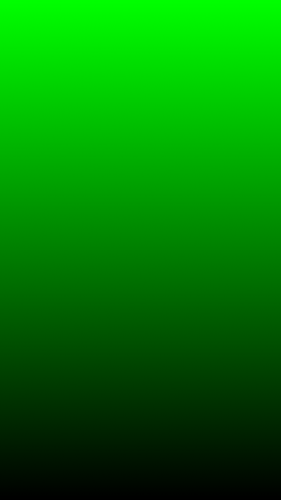Now it’s time for a challenge! See if you can reproduce the screenshot below by just changing one line of code in your shader.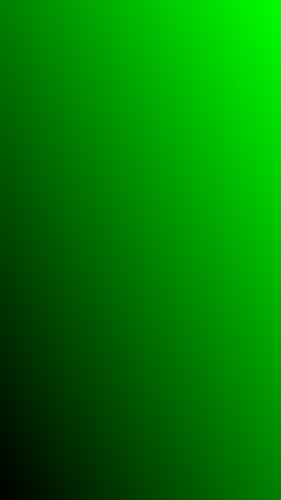Hint: Remember that `position` ranges from `0.0` to `1.0` and so does `gl_FragColor`.

```float gradient = (position.x+position.y)/2.;
```

[/spoiler]

Well done if you figured it out! If you didn’t, just take a moment to review this section again before moving on. :]

In this section, you’ll learn how to use math to draw simple shapes, starting with a 2D disc/circle and finishing with a 3D sphere.

### Geometry: 2D Disc

Open `RWTSphere.fsh` and add the following lines just below precision:

```// Uniforms
uniform vec2 uResolution;
```

This is the same uniform encountered in the previous section and it’s all you’ll need to generate static geometry. To create a disc, add the following lines inside `main(void)`:

```// 1
vec2 center = vec2(uResolution.x/2., uResolution.y/2.);

// 2

// 3
vec2 position = gl_FragCoord.xy - center;

// 4
gl_FragColor = vec4(vec3(0.), 1.);
} else {
gl_FragColor = vec4(vec3(1.), 1.);
}
```

There’s a bit of math here and here are the explanations of what’s happening:

1. The `center` of your disc will be located exactly in the center of your screen.
2. The `radius` of your disc will be half the width of your screen.
3. `position` is defined by the coordinates of the current pixel, offset by the disc center. Think of it as a vector pointing from the center of the disk to the position.
4. `length()` calculates the length of a vector, which in this case is defined by the Pythagorean Theorem `√(position.x²+position.y²)`.
1. If the resulting value is greater than `radius`, then that particular pixel lies outside the disc area and you color it black.
2. Otherwise, that particular pixel lies within the disc and you color it white.

For an explanation of this behavior, look to the circle equation defined as: `(x-a)²+(y-b)² = r²`. Note that `r` is the radius, `ab` is the center and `xy` is the set of all points on the circle.

Since a disc is the region in a plane bounded by a circle, the `if-else` statement will accurately draw a disc in space!

Before you build and run, change your program’s fragment shader source to `RWTSphere` in `RWTViewController.m`:

```self.shader = [[RWTBaseShader alloc] initWithVertexShader:@"RWTBase" fragmentShader:@"RWTSphere"];
```

Now, build and run. Your screen should show a solid white disc with a black background. No, it’s not the most innovative design, but you have to start somewhere.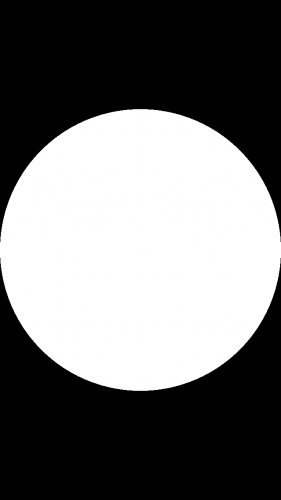Feel free to play around with some of the disc’s properties and see how modifications affect your rendering. For an added challenge, see if you can make the circle shape shown below: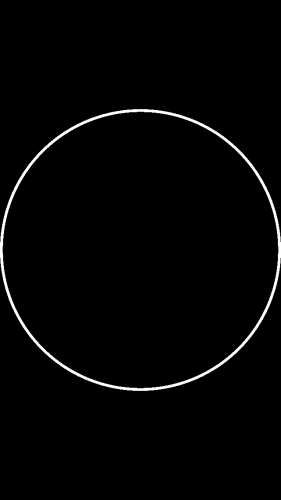Hint: Try creating a new variable called `thickness` defined by your `radius` and used in your `if-else` conditional.
[spoiler title=”Skinny Circle”]

```vec2 center = vec2(uResolution.x/2., uResolution.y/2.);
vec2 position = gl_FragCoord.xy - center;

gl_FragColor = vec4(vec3(0.), 1.);
} else {
gl_FragColor = vec4(vec3(1.), 1.);
}
```

[/spoiler]

If you attempted the challenge or modified your GLSL code, please revert back to that basic solid white disc for now (Kudos for your curiosity though!).

Replace your `if-else` conditional with the following:

```if (length(position) > radius) {
}

gl_FragColor = vec4(vec3(1.), 1.);
```

Dear reader, please let me introduce you to `discard`. `discard` is a fragment-exclusive keyword that effectively tells OpenGL ES to discard the current fragment and ignore it in the following stages of the rendering pipeline. Build and run to see the screen below: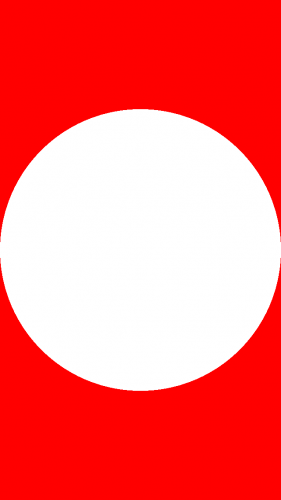In pixel shader terminology, `discard` returns an empty pixel that isn’t written to the screen. Therefore, `glClearColor()` determines the actual screen pixel in its place.

From this point on, when you see a bright red pixel, it means `discard` is working properly. But you should still be wary of a full red screen, as it means something in the code is not right.

### Geometry: 3D Sphere

Now it's time to put a new spin on things and convert that drab 2D disc to a 3D sphere, and to do that you need to account for depth.

In a typical vertex+fragment shader program, this would be simple. The vertex shader could handle 3D geometry input and pass along any information necessary to the fragment shader. However, when working with pixel shaders you only have a 2D plane on which to "paint", so you'll need to fake depth by inferring z values.

Several paragraphs ago you created a disc by coloring any pixels inside a circle defined by:
``` (x-a)²+(y-b)² = r² ```
Extending this to the sphere equation is very easy, like so:
``` (x-a)²+(y-b)²+(z-c)² = r² ```
`c` is the `z` center of the sphere. Since the circle center `ab` offsets your 2D coordinatesand your new sphere will lie on the `z` origin, this equation can be simplified to:
``` x²+y²+z² = r² ```
Solving for `z` results in the equation:
``` z² = √(r²-x²-y²) ```
And that’s how you can infer a `z` value for all fragments, based on their unique position! Luckily enough, this is very easy to code in GLSL. Add the following lines to `RWTSphere.fsh` just before `gl_FragColor`:

```float z = sqrt(radius*radius - position.x*position.x - position.y*position.y);
```

The first line calculates `z` as per your reduced equation, and the second divides by the sphere `radius` to contain the range between `0.0` and `1.0`.

In order to visualize your sphere’s depth, replace your current `gl_FragColor` line with the following:

```gl_FragColor = vec4(vec3(z), 1.);
```

Build and run to see your flat disc now has a third dimension.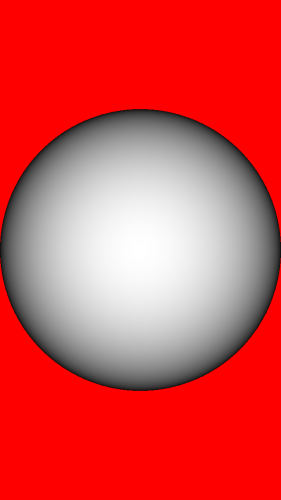Since positive z-values are directed outwards from the screen towards the viewer, the closest points on the sphere are white (middle) while the furthest points are black (edges).

Naturally, any points in between are part of a smooth, gray gradient. This piece of code is a quick and easy way to visualize depth, but it ignores the xy values of the sphere. If this shape were to rotate or sit alongside other objects, you couldn’t tell which way is up/down or left/right.

Replace the line:

```z /= radius;
```

With:

```vec3 normal = normalize(vec3(position.x, position.y, z));
```

A better way to visualize orientation in 3D space is with the use of `normals`. In this example, normals are vectors perpendicular to the surface of your sphere. For any given point, a normal defines the direction that point faces.

In the case of this sphere, calculating the normal for each point is easy. We already have a vector (`position`) that points from the center of the sphere to the current point, as well as its `z` value. This vector doubles as the direction the point is facing, or the normal.

If you’ve worked through some of our previous OpenGL ES tutorials, you know that it's also generally a good idea to `normalize()` vectors, in order to simplify future calculations (particularly for lighting).

Normalized normals lie within the range `-1.0 ≤ n ≤ 1.0`, while pixel color channels lie within the range `0.0 ≤ c ≤ 1.0`. In order to visualize your sphere’s normals properly, define a normal `n` to color `c` conversion like so:

```-1.0 ≤ n ≤ 1.0
(-1.0+1.0) ≤ (n+1.0) ≤ (1.0+1.0)
0.0 ≤ (n+1.0) ≤ 2.0
0.0/2.0 ≤ (n+1.0)/2.0 ≤ 2.0/2.0
0.0 ≤ (n+1.0)/2.0 ≤ 1.0
0.0 ≤ c ≤ 1.0
c = (n+1.0)/2.0
```

Voilà! It's just that simple
Now, replace the line:

```gl_FragColor = vec4(vec3(z), 1.);
```

With:

```gl_FragColor = vec4((normal+1.)/2., 1.);
```

Then build and run. Prepare to feast your eyes on the round rainbow below: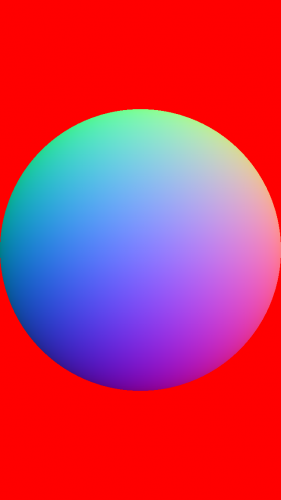This might seem confusing at first, particularly when your previous sphere rendered so smoothly, but there is a lot of valuable information hidden within these colors…

What you're seeing now is essentially a normal map of your sphere. In a normal map, rgb colors represent surface normals which correspond to actual xyz coordinates, respectively. Take a look at the following diagram: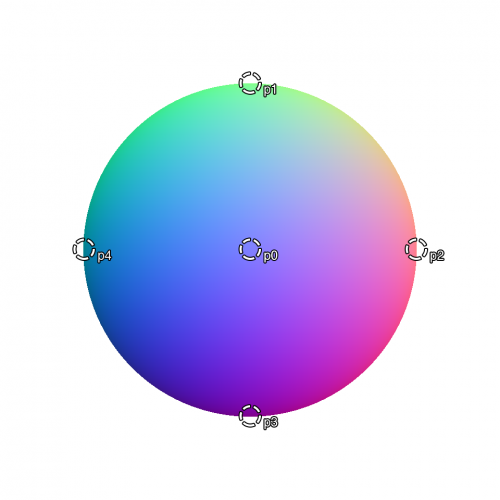The rgb color values for the circled points are:

```p0c = (0.50, 0.50, 1.00)
p1c = (0.50, 1.00, 0.53)
p2c = (1.00, 0.50, 0.53)
p3c = (0.50, 0.00, 0.53)
p4c = (0.00, 0.50, 0.53)
```

Previously, you calculated a normal `n` to color `c` conversion. Using the reverse equation, `n = (c*2.0)-1.0`, these colors can be mapped to specific normals:

```p0n = (0.00, 0.00, 1.00)
p1n = (0.00, 1.00, 0.06)
p2n = (1.00, 0.00, 0.06)
p3n = (0.00, -1.00, 0.06)
p4n = (-1.00, 0.00, 0.06)
```

Which, when represented with arrows, look a bit like this: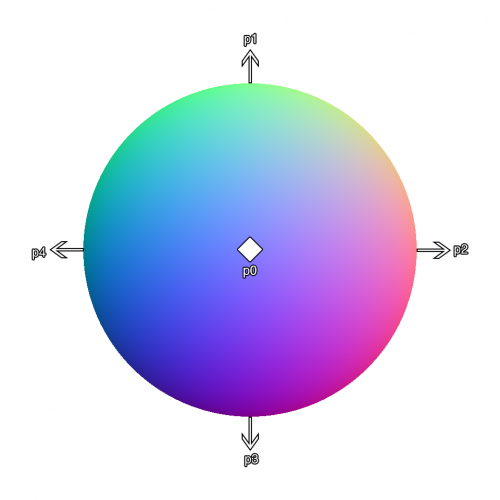Now, there should be absolutely no ambiguity for the orientation of your sphere in 3D space. Furthermore, you can now light your object properly!

Add the following lines above `main(void)` in `RWTSphere.fsh`:

```// Constants
const vec3 cLight = normalize(vec3(.5, .5, 1.));
```

This constant defines the orientation of a virtual light source that illuminates your sphere. In this case, the light gleams towards the screen from the top-right corner.

Next, replace the following line:

```gl_FragColor = vec4((normal+1.)/2., 1.);
```

With:

```float diffuse = max(0., dot(normal, cLight));

gl_FragColor = vec4(vec3(diffuse), 1.);
```

You may recognize this as the simplified `diffuse` component of the Phong reflection model. Build and run to see your nicely-lit sphere!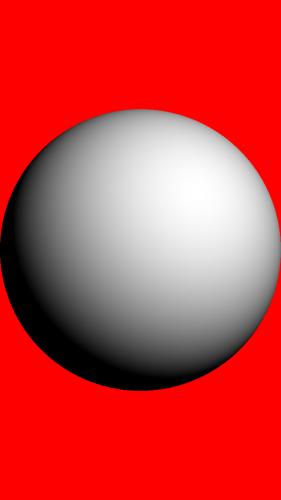Note: To learn more about the Phong lighting model, check out our Ambient, Diffuse, and Specular video tutorials [Subscribers Only].

3D objects on a 2D canvas? Just using math? Pixel-by-pixel? WHOA

This is a great time for a little break so you can bask in the soft, even glow of your shader in all of its glory...and also clear your head a bit because, dear reader, you've only just begun.

## Pixel Shader Procedural Textures: Perlin Noise

In this section, you’ll learn all about texture primitives, pseudorandom number generators, and time-based functions - eventually working your way up to a basic noise shader inspired by Perlin noise.

The math behind Perlin Noise is a bit too dense for this tutorial, and a full implementation is actually too complex to run at 30 FPS.

The basic shader here, however, will still cover a lot of noise essentials (with particular thanks to the modular explanations/examples of Hugo Elias and Toby Schachman).

Ken Perlin developed Perlin noise in 1981 for the movie TRON, and it's one of the most groundbreaking, fundamental algorithms in computer graphics.

It can mimic pseudorandom patterns in natural elements, such as clouds and flames. It is so ubiquitous in modern CGI that Ken Perlin eventually received an Academy Award in Technical Achievement for this technique and its contributions to the film industry.

The award itself explains the gist of Perlin Noise quite nicely:

"To Ken Perlin for the development of Perlin Noise, a technique used to produce natural appearing textures on computer generated surfaces for motion picture visual effects. The development of Perlin Noise has allowed computer graphics artists to better represent the complexity of natural phenomena in visual effects for the motion picture industry."

So yeah, it's kind of a big deal… and you’ll get to implement it from the ground up.

But first, you must familiarize yourself with time inputs and math functions.

### Procedural Textures: Time

Open `RWTNoise.fsh` and add the following lines just below precision highp float;

```// Uniforms
uniform vec2 uResolution;
uniform float uTime;
```

You’re already familiar with the `uResolution` uniform, but `uTime` is a new one. `uTime` comes from the `timeSinceFirstResume` property of your `GLKViewController` subclass, implemented as `RWTViewController.m` (i.e. time elapsed since the first time the view controller resumed update events).

`uTime` handles this time interval in `RWTBaseShader.m` and is assigned to the corresponding GLSL uniform in the method `renderInRect:atTime:`, meaning that `uTime` contains the elapsed time of your app, in seconds.

To see `uTime` in action, add the following lines to `RWTNoise.fsh`, inside `main(void)`:

```float t = uTime/2.;
if (t>1.) {
t -= floor(t);
}

gl_FragColor = vec4(vec3(t), 1.);
```

This simple algorithm will cause your screen to repeatedly fade-in from black to white.

The variable `t` is half the elapsed time and needs converting to fit in between the color range `0.0` to `1.0`. The function `floor()` accomplishes this by returning the nearest integer less than or equal to `t`, which you then subtract from itself.

For example, for `uTime = 5.50:` at `t = 0.75`, your screen will be 75% white.

```t = 2.75
floor(t) = 2.00
t = t - floor(t) = 0.75
```

Before you build and run, remember to change your program’s fragment shader source to `RWTNoise` in `RWTViewController.m`:

```self.shader = [[RWTBaseShader alloc] initWithVertexShader:@"RWTBase" fragmentShader:@"RWTNoise"];
```

Now build and run to see your simple animation!

You can reduce the complexity of your implementation by replacing your `if` statement with the following line:

```t = fract(t);
```

`fract()` returns a fractional value for `t`, calculated as `t - floor(t)`. Ahhh, there, that's much better
Now that you have a simple animation working, it's time to make some noise (Perlin noise, that is).

### Procedural Textures: "Random" Noise

`fract()` is an essential function in fragment shader programming. It keeps all values within `0.0` and `1.0`, and you’ll be using it to create a pseudorandom number generator (PRNG) that will approximate a white noise image.

Since Perlin noise models natural phenomena (e.g. wood, marble), PRNG values work perfectly because they are random-enough to seem natural, but are actually backed by a mathematical function that will produce subtle patterns (e.g. the same seed input will produce the same noise output, every time).

Controlled chaos is the essence of procedural texture primitives!

Note: Computer randomness is a deeply fascinating subject that could easily span dozens of tutorials and extended forum discussions. `arc4random()` in Objective-C is a luxury for iOS developers. You can learn more about it from NSHipster, a.k.a. Mattt Thompson. As he so elegantly puts it, "What passes for randomness is merely a hidden chain of causality".

The PRNG you’ll be writing will be largely based on sine waves, since sine waves are cyclical which is great for time-based inputs. Sine waves are also straightforward as it's just a matter of calling `sin()`.

They are also easy to dissect. Most other GLSL PRNGs are either great, but incredibly complex, or simple, but unreliable.

But first, a quick visual recap of sine waves: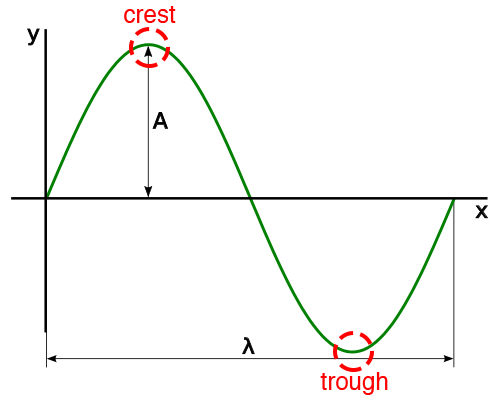You may already be familiar with the amplitude `A` and wavelength `λ`. However, if you're not, don’t worry too much about them; after all, the goal is to create random noise, not smooth waves.

For a standard sine wave, peak-to-peak amplitude ranges from `-1.0` to `1.0` and wavelength is equal to `2π` (frequency = 1).

In the image above, you are viewing the sine wave from the "front", but if you view it from the "top" you can use the waves crests and troughs to draw a smooth greyscale gradient, where crest = white and trough = black.

Open `RWTNoise.fsh` and replace the contents of `main(void)` with the following:

```vec2 position = gl_FragCoord.xy/uResolution.xy;

float pi = 3.14159265359;
float wave = sin(2.*pi*position.x);
wave = (wave+1.)/2.;

gl_FragColor = vec4(vec3(wave), 1.);
```

Remember that `sin(2π)` = 0, so you are multiplying `2π` by the fraction along the x-axis for the current pixel. This way, the far left side of the screen will be the left side of the sin wave, and the far right side of the screen will be the right side of the sin wave.

Also remember the output of `sin` is between -1 and 1, so you add 1 to the result and divide it by 2 to get the output in the range of 0 to 1.

Build and run. You should see a smooth sine wave gradient with one crest and one trough.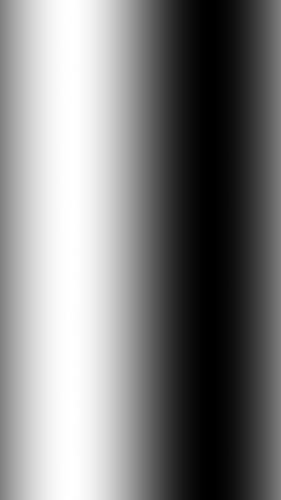Transferring the current gradient to the previous diagram would look something like this: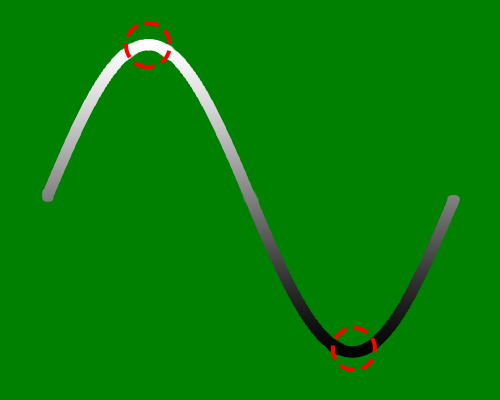Now, make that wavelength shorter by increasing its frequency and factoring in the y-axis of the screen.

Change your `wave` calculation to:

```float wave = sin(4.*2.*pi*(position.x+position.y));
```

Build and run. You should see that your new wave not only runs diagonally across the screen, but also has way more crests and troughs (the new frequency is `4`).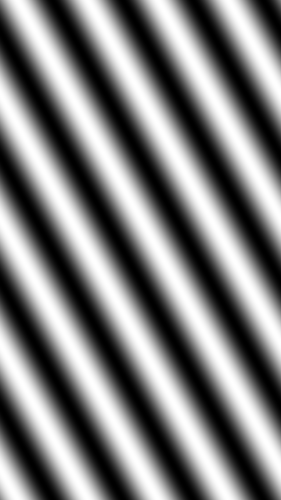So far the equations in your shader have produced neat, predictable results and formed orderly waves. But the goal is entropy, not order, so now it's time to start breaking things a bit. Of course, this is a calm, controlled kind of breaking, not a bull-in-a-china-shop kind of breaking.

Replace the following lines:

```float wave = sin(4.*2.*pi*(position.x+position.y));
wave = (wave+1.)/2.;
```

With:

```float wave = fract(sin(16.*2.*pi*(position.x+position.y)));
```

Build and run. What you’ve done here is increase the frequency of the waves and use `fract()` to introduce harder edges in your gradient. You're also no longer performing a proper conversion between different ranges, which adds a bit of spice in the form of chaos.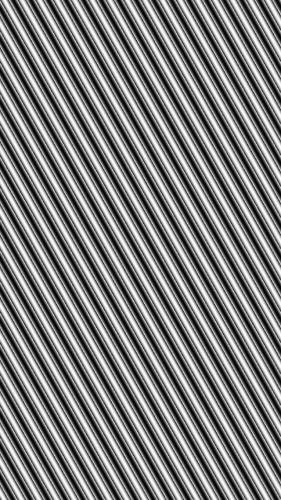The pattern generated by your shader is still fairly predictable, so go ahead and throw another wrench in the gears.

Change your `wave` calculation to:

```float wave = fract(10000.*sin(16.*(position.x+position.y)));
```

Now build and run to see a salt & pepper spill.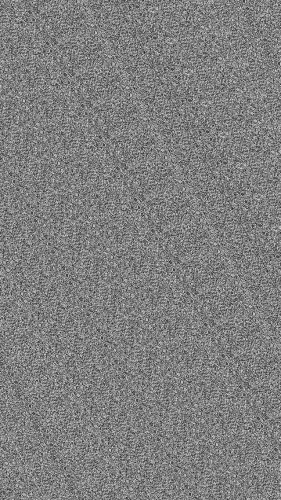The `10000` multiplier is great for generating pseudorandom values and can be quickly applied to sine waves using the following table:

```Angle sin(a)
1.0   .0174
2.0   .0349
3.0   .0523
4.0   .0698
5.0   .0872
6.0   .1045
7.0   .1219
8.0   .1392
9.0   .1564
10.0  .1736
```

Observe the sequence of numbers for the second decimal place:

```1, 3, 5, 6, 8, 0, 2, 3, 5, 7
```

Now observe the sequence of numbers for the fourth decimal place:

```4, 9, 3, 8, 2, 5, 9, 2, 4, 6
```

A pattern is more apparent in the first sequence, but less so in the second. While this may not always be the case, less significant decimal places are a good starting place for mining pseudorandom numbers.

It also helps that really large numbers may have unintentional precision loss/overflow errors.

At the moment, you can probably still see a glimpse of a wave imprinted diagonally on the screen. If not, it might be time to pay a visit to your optometrist. ;]

The faint wave is simply a product of your calculation giving equal importance to `position.x` and `position.y` values. Adding a unique multiplier to each axis will dissipate the diagonal print, like so:

```float wave = fract(10000.*sin(128.*position.x+1024.*position.y));
```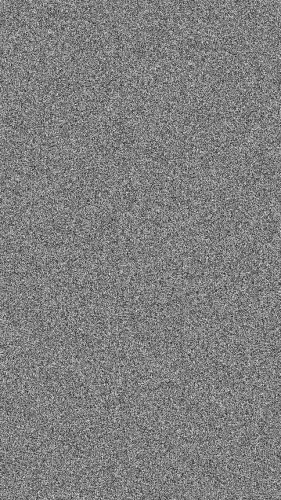Time for a little clean up! Add the following function, `randomNoise(vec2 p)`, above `main(void)`:

```float randomNoise(vec2 p) {
return fract(6791.*sin(47.*p.x+p.y*9973.));
}
```

I chose the ones above from a list of prime numbers and you can use it too. If you select your own numbers, I would recommend a small value for `p.x`, and larger ones for `p.y` and `sin()`.

Next, refactor your shader to use your new `randomNoise` function by replacing the contents of `main(void)` with the following:

```vec2 position = gl_FragCoord.xy/uResolution.xy;
float n = randomNoise(position);
gl_FragColor = vec4(vec3(n), 1.);
```

Presto! You now have a simple sin-based PRNG for creating 2D noise. Build and run, then take a break to celebrate, you've earned it.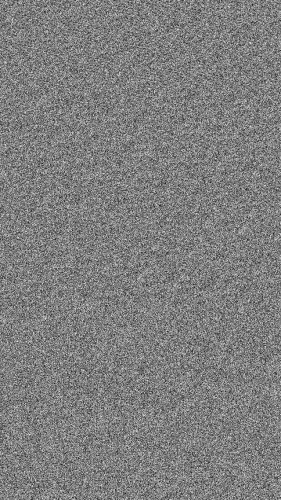### Procedural Textures: Square Grid

When working with a 3D sphere, normalizing vectors makes equations much simpler, and the same is true for procedural textures, particularly noise. Functions like smoothing and interpolation are a lot easier if they happen on a square grid. Open `RWTNoise.fsh` and replace the calculation for `position` with this:

```vec2 position = gl_FragCoord.xy/uResolution.xx;
```

This ensures that one unit of `position` is equal to the width of your screen (`uResolution.x`).

On the next line, add the following if statement:

```if ((position.x>1.) || (position.y>1.)) {
}
```

Make sure you give `discard` a warm welcome back into you code, then build and run to render the image below: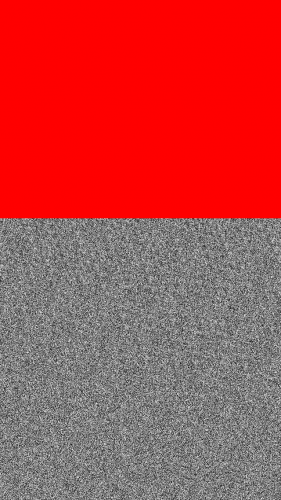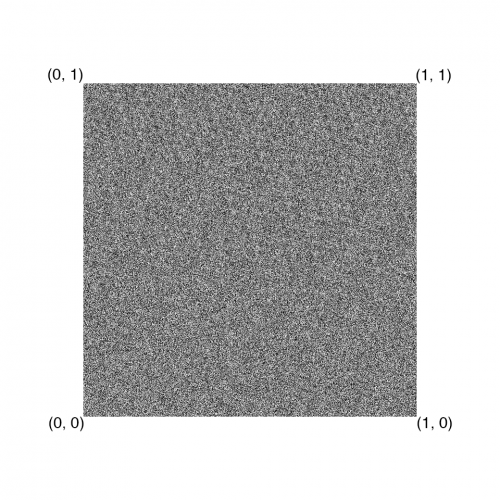Since 2D noise extends infinitely in x and y, if you replace your noise input with either of the following lines below:

```float n = randomNoise(position-1.);
float n = randomNoise(position+1.);
```

This is what you'll see:

For any noise-based procedural texture, there is a primitive-level distinction between too much noise and not enough noise. Fortunately, tiling your square grid makes it possible to control this.

Add the following lines to `main(void)`, just before `n`:

```float tiles = 2.;
position = floor(position*tiles);
```

Then build and run! You should see a 2x2 square grid like the one below: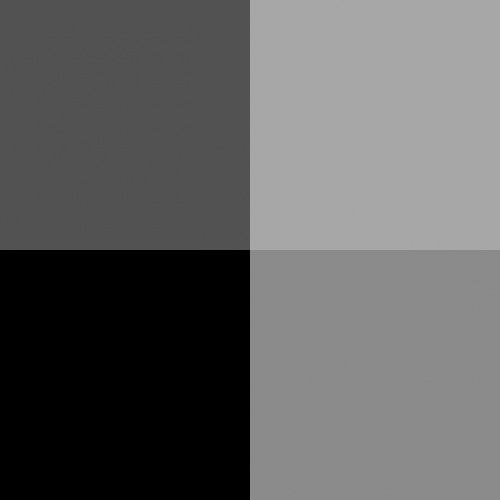This might be a bit confusing at first, so here's an explanation: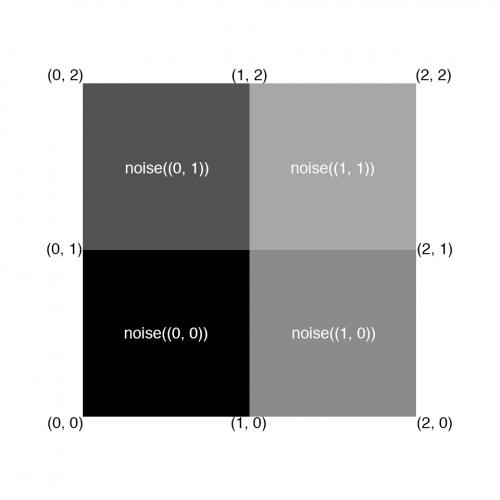`floor(position\*tiles)` will truncate any value to the nearest integer less than or equal to `position*tiles`, which lies in the range `(0.0, 0.0)` to `(2.0, 2.0)`, in both directions.

Without `floor()`, this range would be continuously smooth and every fragment position would seed `noise()` with a different value.

However, `floor()` creates a stepped range with stops at every integer, as shown in the diagram above. Therefore, every `position` value in-between two integers will be truncated before seeding `noise()`, creating a nicely-tiled square grid.

The number of square tiles you choose will depend on the type of texture effect you want to create. Perlin noise adds many grids together to compute its noisy pattern, each with a different number of tiles.

There is such a thing as too many tiles, which often results in blocky, repetitive patterns. For example, the square grid for `tiles = 128.` looks something like this: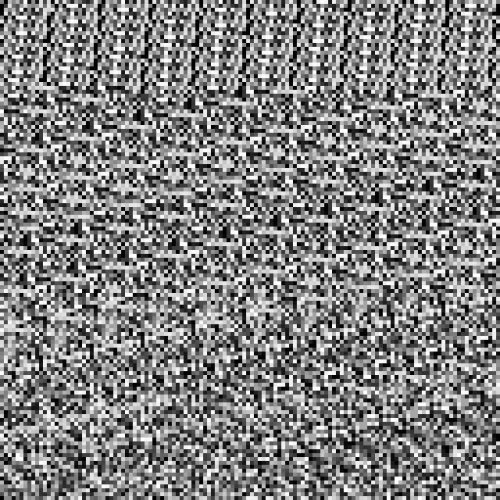### Procedural Textures: Smooth Noise

At the moment, your noise texture is a bit too, ahem, noisy. This is good if you wish to texture an old-school TV set with no signal, or maybe MissingNo.

But what if you want a smoother texture? Well, you would use a smoothing function. Get ready for a shift gears and move onto image processing 101.

In 2D image processing, pixels have a certain connectivity with their neighbors. An 8-connected pixel has eight neighbors surrounding it; four touching at the edges and four touching at the corners.

You might also know this concept as a Moore neighborhood and it looks something like this, where CC is the centered pixel in question: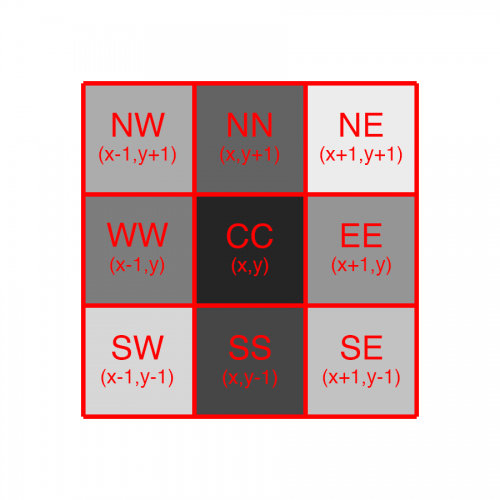Note: To learn more about the Moore neighborhood and image processing in general, check out our Image Processing in iOS tutorial series.

A common use of image smoothing operations is attenuating edge frequencies in an image, which produces a blurred/smeared copy of the original. This is great for your square grid because it reduces harsh intensity changes between neighboring tiles.

For example, if white tiles surround a black tile, a smoothing function will adjust the tiles' color to a lighter gray. Smoothing functions apply to every pixel when you use a convolution kernel, like the one below: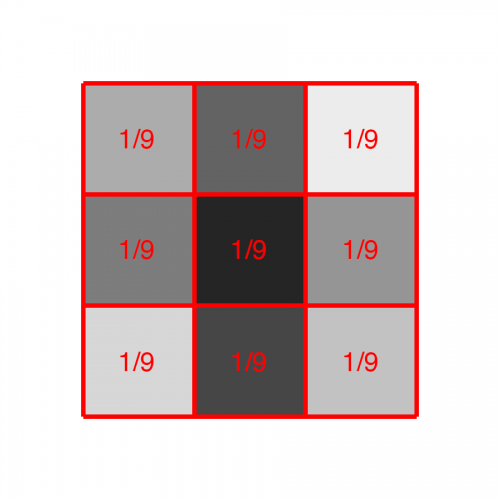This is a 3x3 neighborhood averaging filter, which simply smooths a pixel value by averaging the values of its 8 neighbors (with equal weighting). To produce the image above, this would be the code:

```p = 0.1
p’ = (0.3+0.9+0.5+0.7+0.2+0.8+0.4+0.6+0.1) / 9
p’ = 4.5 / 9
p’ = 0.5
```

It’s not the most interesting filter, but it’s simple, effective and easy to implement! Open `RWTNoise.fsh` and add the following function just above `main(void)`:

```float smoothNoise(vec2 p) {
vec2 nn = vec2(p.x, p.y+1.);
vec2 ne = vec2(p.x+1., p.y+1.);
vec2 ee = vec2(p.x+1., p.y);
vec2 se = vec2(p.x+1., p.y-1.);
vec2 ss = vec2(p.x, p.y-1.);
vec2 sw = vec2(p.x-1., p.y-1.);
vec2 ww = vec2(p.x-1., p.y);
vec2 nw = vec2(p.x-1., p.y+1.);
vec2 cc = vec2(p.x, p.y);

float sum = 0.;
sum += randomNoise(nn);
sum += randomNoise(ne);
sum += randomNoise(ee);
sum += randomNoise(se);
sum += randomNoise(ss);
sum += randomNoise(sw);
sum += randomNoise(ww);
sum += randomNoise(nw);
sum += randomNoise(cc);
sum /= 9.;

return sum;
}
```

It’s a bit long, but also pretty straightforward. Since your square grid is divided into 1x1 tiles, a combination of `±1.` in either direction will land you on a neighboring tile. Fragments are batch-processed in parallel by the GPU, so the only way to know about neighboring fragment values in procedural textures is to compute them on the spot.

Modify `main(void)` to have 128 `tiles`, and compute `n` with `smoothNoise(position)`. After those changes, your `main(void)` function should look like this:

```void main(void) {
vec2 position = gl_FragCoord.xy/uResolution.xx;
float tiles = 128.;
position = floor(position*tiles);
float n = smoothNoise(position);
gl_FragColor = vec4(vec3(n), 1.);
}
```

Build and run! You’ve been hit by, you’ve been struck by, a smooooooth functional. :P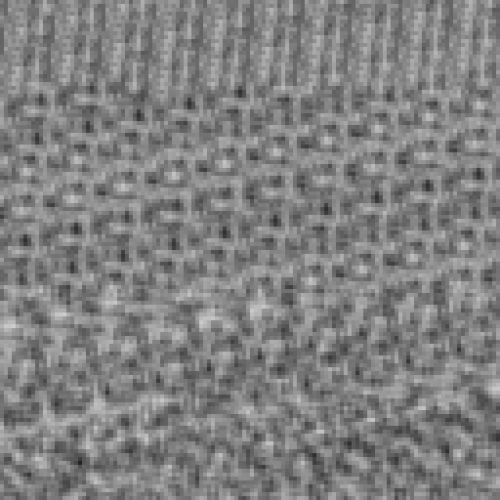Nine separate calls to `randomNoise()`, for every pixel, are quite the GPU load. It doesn't hurt to explore 8-connected smoothing functions, but you can produce a pretty good smoothing function with 4-connectivity, also called the Von Neumann neighborhood.

Neighborhood averaging also produces a rather harsh blur, turning your pristine noise into grey slurry. In order to preserve original intensities a bit more, you’ll implement the convolution kernel below: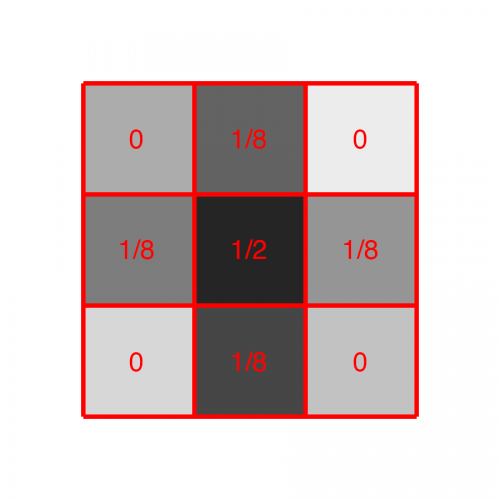This new filter reduces neighborhood averaging significantly by having the pixel in question contribute 50% of the final result, with the other 50% coming from its 4 edge-neighbors. For the image above, this would be:

```p = 0.1
p’ = (((0.3+0.5+0.2+0.4) / 4) / 2) + (0.1 / 2)
p’ = 0.175 + 0.050
p’ = 0.225
```

Time for a quick challenge! See if you can implement this half-neighbor-averaging filter in `smoothNoise(vec2 p)`.

Hint: Remember to remove any unnecessary neighbors! Your GPU will thank you and reward you with faster rendering and less griping.

[spoiler title="Smooth Noise Filter"]

```float smoothNoise(vec2 p) {
vec2 nn = vec2(p.x, p.y+1.);
vec2 ee = vec2(p.x+1., p.y);
vec2 ss = vec2(p.x, p.y-1.);
vec2 ww = vec2(p.x-1., p.y);
vec2 cc = vec2(p.x, p.y);

float sum = 0.;
sum += randomNoise(nn)/8.;
sum += randomNoise(ee)/8.;
sum += randomNoise(ss)/8.;
sum += randomNoise(ww)/8.;
sum += randomNoise(cc)/2.;

return sum;
}
```

[/spoiler]

If you didn't figure it out, take a look at the code in the spoiler, and replace your existing `smoothNoise` method with it. Reduce your number of `tiles` to `8.`, then build and run.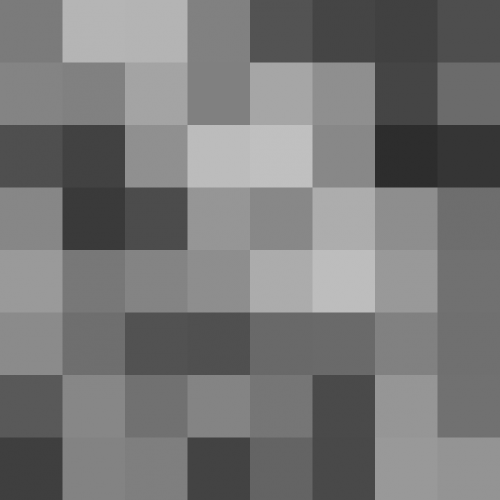Your texture is starting to look more natural, with smoother transitions between tiles. Compare the image above (smooth noise) with the one below (random noise) to appreciate the impact of the smoothing function.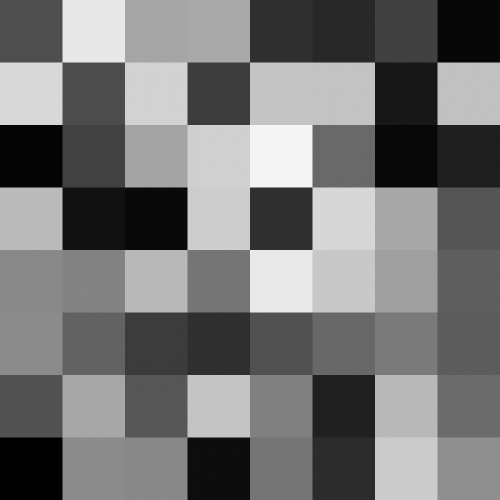Great job so far :]

### Procedural Textures: Interpolated Noise

The next step for your noise shader is rid the tiles of hard edges by using bilinear interpolation, which is simply linear interpolation on a 2D grid.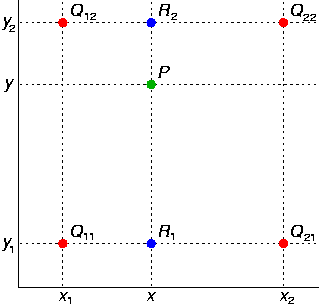For ease of comprehension, the image below shows the desired sampling points for bilinear interpolation within your noise function roughly translated to your previous 2x2 grid: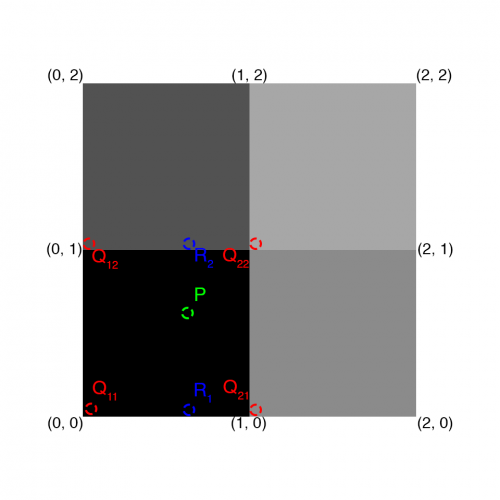Tiles can blend into one another by sampling weighted values from their corners at point `P`. Since each tile is 1x1 unit, the `Q` points should be sampling noise like so:

```Q11 = smoothNoise(0.0, 0.0);
Q12 = smoothNoise(0.0, 1.0);
Q21 = smoothNoise(1.0, 0.0);
Q22 = smoothNoise(1.0, 1.0);
```

In code, you achieve this with a simple combination of `floor()` and `ceil()` functions for `p`. Add the following function to `RWTNoise.fsh`, just above `main(void)`:

```float interpolatedNoise(vec2 p) {
float q11 = smoothNoise(vec2(floor(p.x), floor(p.y)));
float q12 = smoothNoise(vec2(floor(p.x), ceil(p.y)));
float q21 = smoothNoise(vec2(ceil(p.x), floor(p.y)));
float q22 = smoothNoise(vec2(ceil(p.x), ceil(p.y)));

// compute R value
// return P value
}
```

GLSL already includes a linear interpolation function called `mix()`.

You'll use it to compute `R1` and `R2`, using `fract(p.x)` as the weight between two `Q` points at the same height on the y-axis. Include this in your code by adding the following lines at the bottom of `interpolatedNoise(vec2 p)`:

```float r1 = mix(q11, q21, fract(p.x));
float r2 = mix(q12, q22, fract(p.x));
```

Finally, interpolate between the two `R` values by using `mix()` with `fract(p.y)` as the floating-point weight. Your function should look like the following:

```float interpolatedNoise(vec2 p) {
float q11 = smoothNoise(vec2(floor(p.x), floor(p.y)));
float q12 = smoothNoise(vec2(floor(p.x), ceil(p.y)));
float q21 = smoothNoise(vec2(ceil(p.x), floor(p.y)));
float q22 = smoothNoise(vec2(ceil(p.x), ceil(p.y)));

float r1 = mix(q11, q21, fract(p.x));
float r2 = mix(q12, q22, fract(p.x));

return mix (r1, r2, fract(p.y));
}
```

Since your new function requires smooth, floating-point weights and implements `floor()` and `ceil()` for sampling, you must remove `floor()` from `main(void)`.

Replace the lines:

```float tiles = 8.;
position = floor(position*tiles);
float n = smoothNoise(position);
```

With the following:

```float tiles = 8.;
position *= tiles;
float n = interpolatedNoise(position);
```

Build and run. Those hard tiles are gone…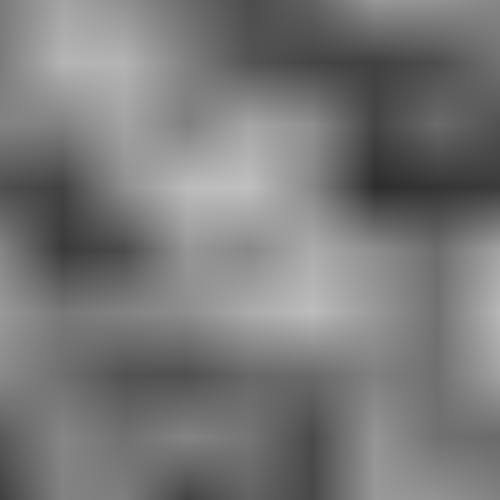… but there is still a discernible pattern of "stars", which is totally expected, by the way.

You’ll get rid of the undesirable pattern with a smoothstep function. `smoothstep()` is a nicely curved function that uses cubic interpolation, and it's much nicer than simple linear interpolation.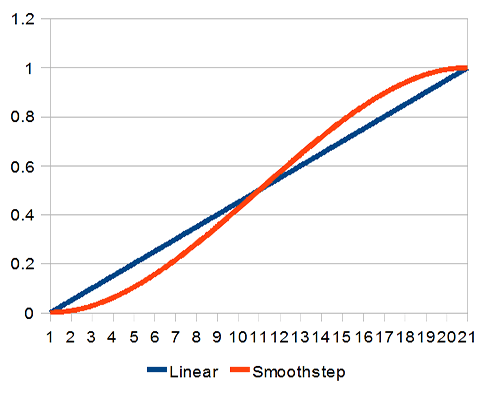"Smoothstep is the magic salt you can sprinkle over everything to make it better." --Jari Komppa

Add the following line inside `interpolatedNoise(vec2 p)`, at the very beginning:

```vec2 s = smoothstep(0., 1., fract(p));
```

Now you can use `s` as the smooth-stepped weight for your `mix()` functions, like so:

```float r1 = mix(q11, q21, s.x);
float r2 = mix(q12, q22, s.x);

return mix (r1, r2, s.y);
```

Build and run to make those stars disappear!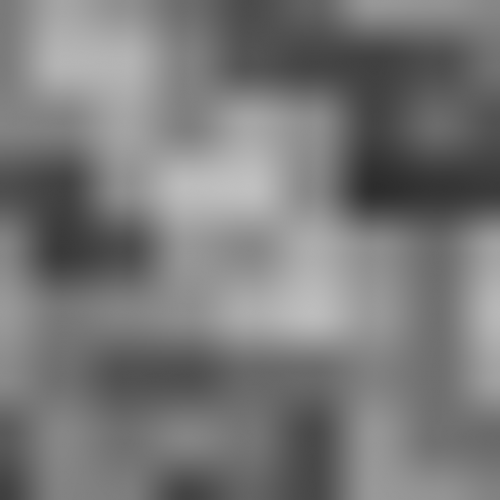The stars are definitely gone, but there’s still a bit of a pattern; almost like a labyrinth. This is simply due to the 8x8 dimensions of your square grid. Reduce `tiles` to `4.`, then build and run again!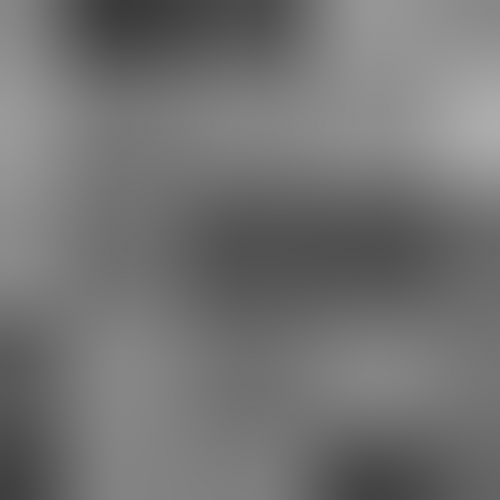Much better.
Your noise function is still a bit rough around the edges, but it could serve as a texture primitive for billowy smoke or blurred shadows.

### Procedural Textures: Moving Noise

Final stretch! Hope you didn’t forget about little ol' `uTime`, because it’s time to animate your noise. Simply add the following line inside `main(void)`, just before assigning `n`:

```position += uTime;
```

Build and run.

Your noisy texture will appear to be moving towards the bottom-left corner, but what’s really happening is that you’re moving your square grid towards the top-right corner (in the +x, +y direction). Remember that 2D noise extends infinitely in all directions, meaning your animation will be seamless at all times.

Hypothesis: Sphere + Noise = Moon? You're about to find out!
To wrap up this tutorial, you’ll combine your sphere shader and noise shader into a single moon shader in RWTMoon.fsh. You have all the information you need to do this, so this is a great time for a challenge!

Hint: Your noise `tiles` will now be defined by the sphere’s `radius`, so replace the following lines:

```float tiles = 4.;
position *= tiles;
```

With a simple:

```position /= radius;
```

Also, I double-dare you to refactor a little bit by completing this function:

```float diffuseSphere(vec2 p, float r) {
}
```

[spoiler title="Werewolves, Beware"]

```//  RWTMoon.fsh
//
// Precision
precision highp float;

// Uniforms
uniform vec2 uResolution;
uniform float uTime;

// Constants
const vec3 cLight = normalize(vec3(.5, .5, 1.));

float randomNoise(vec2 p) {
return fract(6791.*sin(47.*p.x+p.y*9973.));
}

float smoothNoise(vec2 p) {
vec2 nn = vec2(p.x, p.y+1.);
vec2 ee = vec2(p.x+1., p.y);
vec2 ss = vec2(p.x, p.y-1.);
vec2 ww = vec2(p.x-1., p.y);
vec2 cc = vec2(p.x, p.y);

float sum = 0.;
sum += randomNoise(nn)/8.;
sum += randomNoise(ee)/8.;
sum += randomNoise(ss)/8.;
sum += randomNoise(ww)/8.;
sum += randomNoise(cc)/2.;

return sum;
}

float interpolatedNoise(vec2 p) {
vec2 s = smoothstep(0., 1., fract(p));

float q11 = smoothNoise(vec2(floor(p.x), floor(p.y)));
float q12 = smoothNoise(vec2(floor(p.x), ceil(p.y)));
float q21 = smoothNoise(vec2(ceil(p.x), floor(p.y)));
float q22 = smoothNoise(vec2(ceil(p.x), ceil(p.y)));

float r1 = mix(q11, q21, s.x);
float r2 = mix(q12, q22, s.x);

return mix (r1, r2, s.y);
}

float diffuseSphere(vec2 p, float r) {
float z = sqrt(r*r - p.x*p.x - p.y*p.y);
vec3 normal = normalize(vec3(p.x, p.y, z));
float diffuse = max(0., dot(normal, cLight));
return diffuse;
}

void main(void) {
vec2 center = vec2(uResolution.x/2., uResolution.y/2.);
vec2 position = gl_FragCoord.xy - center;

}

// Diffuse

// Noise
position += uTime;
float noise = interpolatedNoise(position);

gl_FragColor = vec4(vec3(diffuse*noise), 1.);
}
```

[/spoiler]

Remember to change your program’s fragment shader source to `RWTMoon` in `RWTViewController.m`:

```self.shader = [[RWTBaseShader alloc] initWithVertexShader:@"RWTBase" fragmentShader:@"RWTMoon"];
```

While you’re there, feel free to change your `glClearColor()` to complement the scene a bit more (I chose xkcd’s midnight purple):

```glClearColor(.16f, 0.f, .22f, 1.f);
```

Build and run! Oh yeah, I’m sure Ozzy Osbourne would approve.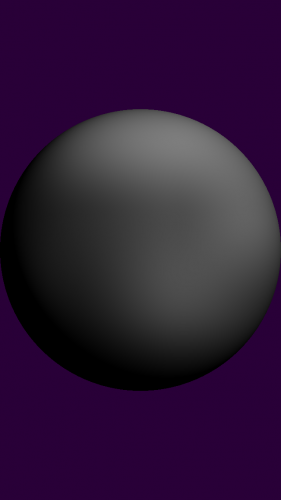## Where To Go From Here?

Here is the completed project with all of the code and resources for this OpenGL ES Pixel Shaders tutorial. You can also find its repository on GitHub.

Congratulations, you've taken a very deep dive into shaders and GPUs, like a daring math-stronaut, testing all four dimensions, as well as the limits of iOS development itself! This was quite a different and difficult tutorial, so I whole-heartedly applaud your efforts.

You should now understand how to use the immense power of the GPU, combined with clever use of math, to create interesting pixel-by-pixel renderings. You should be comfortable with GLSL functions, syntax and organization too.

There wasn't much Objective-C in this tutorial, so feel free to go back to your CPU and think of cool ways to manipulate your shaders even more!

Try adding uniform variables for touch points, or gyroscope data, or microphone input. Browser + WebGL may be more powerful, but Mobile + OpenGL ES is certainly be more interesting :]

There are many paths to explore from here on out, and here are a few suggestions:

• Want to make your shaders super performant? Check out Apple's OpenGL ES tuning tips (highly recommended for iPhone 5s).
• Want to read up on Perlin noise and complete your implementation? Please check-in with Ken himself for a quick presentation or detailed history.
• Think you need to practice the basics? Toby's got just the thing.
• Or maybe, just maybe, you think you're ready for the pros? Well then, please see the masters at work on Shadertoy and drop us a line in the comments if you make any contributions!

In general, I suggest you check out the amazing GLSL Sandbox gallery straight away.

There you can find shaders for all levels and purposes, plus the gallery is edited/curated by some of the biggest names in WebGL and OpenGL ES. They're the rockstars that inspired this tutorial and are shaping the future of 3D graphics, so a big THANKS to them. (Particularly @mrdoob, @iquilezles, @alteredq.)

If you have any questions, comments or suggestions, feel free to join the discussion below!

5/5

3 ratings